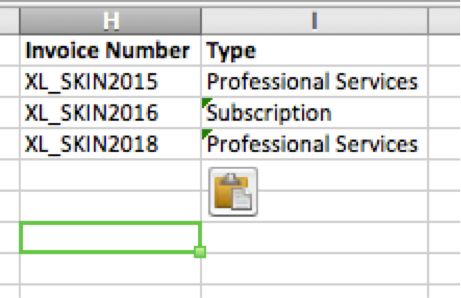# The Excel Skinny

A blog about Excel and its users

## Find the closest match with the VLOOKUP function

April 30, 2017

In previous blog posts we learned how to use the VLOOKUP and HLOOKUP functions to find exact matches in a table. Today we are going to learn how to use the VLOOKUP function to find the closest match, instead of an exact match.

This is accomplished by changing the last part of the formula syntax, [range_lookup].  Setting this argument to FALSE returns only exact matches, but if set to TRUE, the returned value of the function will be the closest match.  Note, the table you are pulling data from must be in ascending order for the closest match to work correctly.

When would you want the closest match rather than an exact match?  Calculating grades is a great example for the closest match VLOOKUP. Keep in mind is the VLOOKUP matches the value that is less than or equal to the lookup value.So we have a score of 81, but we want to know the actual grade:This searches the Scale table and finds that 81 is closest to a C, so it returns the letter C in the cell that we added our formula.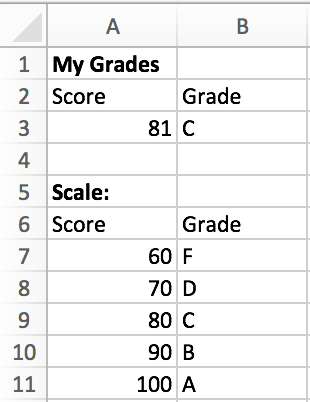And that's all there is to it!  Now you can use the VLOOKUP function to search for exact matches, or closest matches in your spreadsheet.

As always, please let us know if you have any questions!

April 30, 2017

## VLOOKUP on Mac Excel

The VLOOKUP function, when mastered, is one of the most useful functions in Microsoft Excel.  A VLOOKUP is a function that works off the first column in a list of data.  When would you use a VLOOKUP?  When you are trying to pull specific data from a list into another cell.  For our example we will use an Invoice List: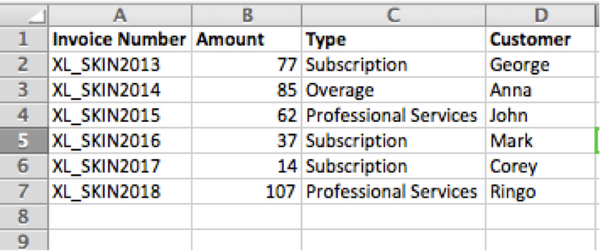For a VLOOKUP to work you must have a unique identifier and that unique identifier must be in the first column of your list.  In this example our unique identifier is the Invoice Number.  Once the VLOOKUP is executed if we put XL_SKIN2013 in a cell with the function it will return to us all the information we want.  Please note the VLOOKUP function has no restrictions whether you want to pull information into the same spreadsheet, same workbook, or different workbooks.

To start, we put our Unique Identifier into a new cell.  This will be the new list where we want to pull this information into.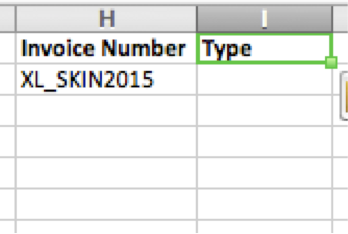The cell next to XL_SKIN2015 will be where we enter the VLOOKUP formula.  Refer back to the Formula Builder Shortcut to open the Formula Builder.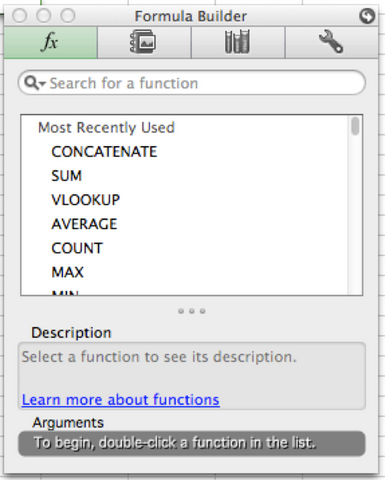Type VLOOKUP in the Search for a Function and double click VLOOKUP to start the function: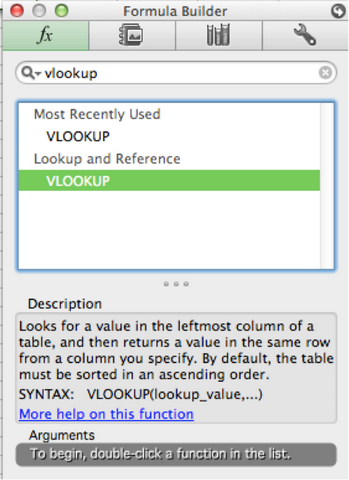The formula builder will ask you for lookup_value, table_array, col_index_num, and range_lookup(optional).  For these values insert:

• Lookup_value = the value to be found in the first column of the table, and can be a value, a reference, or a text string.
• If you have you click the white box next to the lookup_value you can then go to your new list (where you will be pulling information into) and select the Unique Identifier you have already inserted:• Table_array = Tell the VLOOKUP where the original database/list is.  Select the entire list for this. Do not include headers.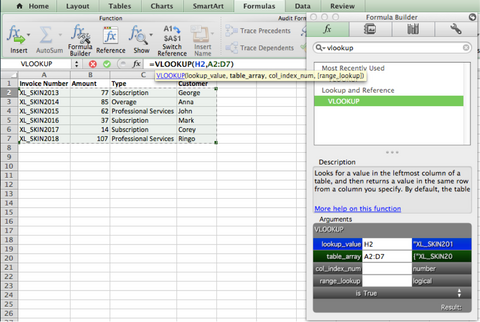• Col_index_num = this part of the VLOOKUP is to specify which information from the original database or list we want.  In our second list we said we want the Type of invoice, so we put 3 for Column 3.  Please note the col_index_num is always the number of columns in from your database or list.  It does not matter where the list starts or ends in relation to the Columns and Rows laid out from Microsoft Excel.
• Range_Lookup = This is either True or False.  Use the following parameters to decide if you need a value in the Range_Lookup:
• If the first column of the database (the column that contains the unique identifiers) is sorted alphabetically/numerically in ascending order, then it’s possible to enter a value of true into this argument, or leave it blank.
• If the first column of the database is not sorted, or it’s sorted in descending order, then you must enter a value of false into this argument
• Our first column in the database is sorted so we do not need a value here.

Press Enter and the information should appear in the VLOOKUP cell!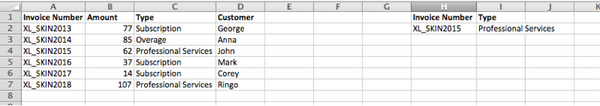If you would like to add more information to the new list simply drag the VLOOKUP down and keep adding Invoice Numbers.  The information will automatically populate: